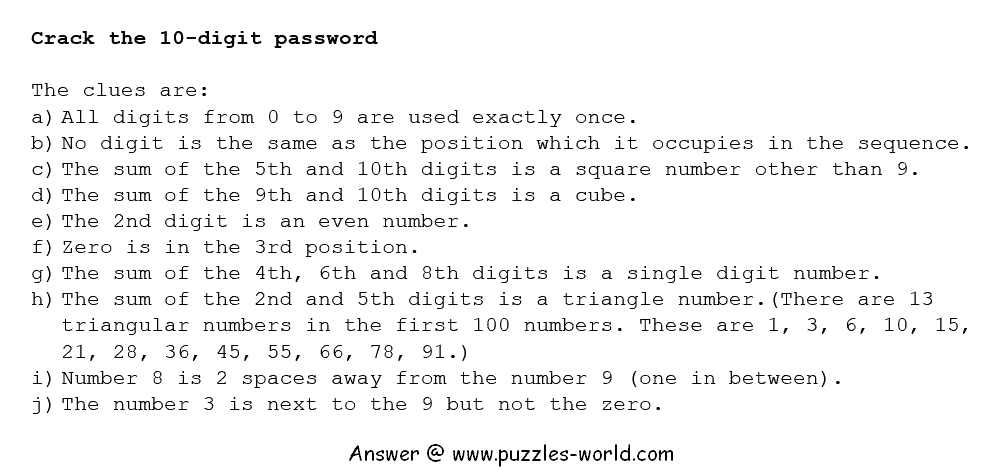The clues are:
a) All digits from 0 to 9 are used exactly once.
b) No digit is the same as the position which it occupies in the sequence.
c) The sum of the 5th and 10th digits is a square number other than 9.
d) The sum of the 9th and 10th digits is a cube.
e) The 2nd digit is an even number.
f) Zero is in the 3rd position.
g) The sum of the 4th, 6th and 8th digits is a single digit number.
h) The sum of the 2nd and 5th digits is a triangle number.(There are 13 triangular numbers in the first 100 numbers. These are 1, 3, 6, 10, 15, 21, 28, 36, 45, 55, 66, 78, 91.)
i) Number 8 is 2 spaces away from the number 9 (one in between).
j) The number 3 is next to the 9 but not the zero.

Share with your friends and family and see how many can solve the puzzle and find the password.

Explanation Steps : Respective Clues are in ().

1] Since, every digit is used only once (1), the sum of 2 digits can't exceed 9 + 8 = 17 and minimum sum of 2 digits can be only 0 + 1 = 1.

2] So, the sum of 5th & 10th digits which is square (3) can be - 1, 4, 16.
The sum of 9th & 10th digits which is cube (4) can be - 1, 8.
The sum of 2nd & 5th digits which is triangle number (8) can be - 1, 3, 6, 10, 15.

3] As per (6), 0 is already at third position i.e. C = 0. Hence, 2nd, 5th, 9th or 10th digits can't be 0. Therefore, the sum of 5th & 10th or 9th & 10th or 2nd & 5th can't be 1. Revising list of possible values of those in step 2.

The sum of 5th & 10th digits which is square (3) can be - 4, 16.
The sum of 9th & 10th digits which is cube (4) can be - 8.
The sum of 2nd & 5th digits which is triangle number (8) can be - 3, 6, 10, 15.

4] Possible pairs of 9th & 10th digits - (1,7), (7,1), (2,6), (6,2), (3,5), (5,3)

If IJ = 71, then 5th digit E must be 4 - 1 = 3 & possible values of 2nd digit B are 0,3,7.
But as per (5), B must be even & C = 0 already.

IJ = 26 or 62 or 35 doesn't leave any valid value for 5th digit E.

If IJ = 53, then 5th digit E must be 1 & possible values of 2nd digit B are 2,5,9
That is B = 2 (5). But as per (2), no digit is the same as the position which it occupies in the sequence. So, the number 2 can't be at 2nd position where B is there.

Hence, IJ must be 17 i.e. I = 1, J = 7.

5] This leaves only 9 as valid value for 5th digit E so that (3) is true.

So, E = 9 and hence B = 6 with sum of 2nd digit B and 5th digit E as 15.

6] So far, we have, B = 6, C = 0, E = 9, I = 1 and J = 7.

As per (10), the number 3 is next to the 9 but not the zero. Hence, the 6th digit F must be 3. F = 3.

7] As per (9), the 8 is 2 spaces away from 9 i.e. here 5th digit E=9. With 3rd position already occupied by C = 0, the digit 8 must be at 7th position.
So, G = 8.

8] As per (7), D + F + H must be single digit. We have, F = 3 already,
so possible values of D + H are 0, 1, 2, 3, 4, 5, 6.

D + H can't be 0 (0+0), 1 (1+0), 2(1+1/2+0), 3(1+2/3+0), 4(2+2/3+1/4+0), 5(2+3/4+1/5+0) since digits are repeated with C=0, I=1, F=3 already.

Hence, D + H = 6.

9] Now, D = 5 and H = 1 is not possible. Also, D/H can't be 0 or 6. Both D and H can't be 3 at the same time. D can't be 4 as D is at 4th position.

Hence, D = 2, H = 4.

10] With B = 6, C = 0, D = 2, E = 9, F = 3, G = 8, H = 4, I = 1, J = 7, the only digit left for the first position A is 5. So A = 5.

CONCLUSION :

A = 5, B = 6, C = 0, D = 2, E = 9, F = 3, G = 8, H = 4, I = 1, J = 7.

So, the 10-digits password ABCDEFGHIJ is 5602938417.